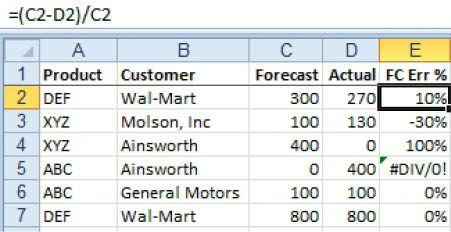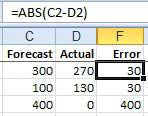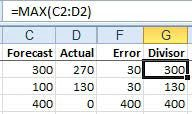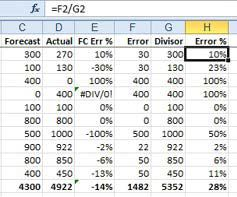Risk Management

# Excel Helps You See The Future

Measure the accuracy of a sales forecast.

A reader posed the following question: “I handle forecasting for my company. I collect forecasts from the sales reps and attempt to turn them into a production plan for the manufacturing plant. Can Excel help me with this chore?”A lot of forecasting professionals measure forecast error as (Forecast-Actual)/Forecast (see Figure 1 at left).

However, there are two kinds of problems in forecasting. If you forecast 400 units and the order does not show up, then the manufacturing plant has 400 sets of material on hand and nowhere to send them. Inventory goes up. This is bad. On the other side, if you forecast 0 units and an order for 400 shows up, the plant has to scramble and start buying material on the gray market. This means the product cost could double and your profits go away. This is also bad.You need a formula for forecast accuracy that treats both of these situations as equally bad. You take the absolute value of (Forecast-Actual) and divide by the larger of the forecasts or actuals. To calculate forecast accuracy using my formula, you follow these steps:

1). Whether the forecast was high or low, the error is always a positive number, so calculate the absolute error on a product-by-product basis. Use the ABS function to return the absolute value of a number (see Figure 2, above left).2). Calculate the divisor (which is what I call the “Size of the opportunity to mess up”). Missing a 1,000-unit sale is much worse than missing a 2-unit sale. For column G, use the MAX function to find what is larger: forecast or actuals (see Figure 3 at right).

3). Calculate the error percentage by dividing F2/G2 (see Figure 4, below left). As shown above, the traditional forecast error calculation is in E. The forecast error calculation you just did is in H. Sometimes these two calculations are the same. Overall, though, because my calculation takes into account the negative effect of an unforecasted order showing up, my error percentage will be higher (and, I feel, more meaningful).This started out as a topic on using ABS and MAX functions but turned into a sermon on the best way to calculate forecast accuracy. Note that I am currently the only person I know who calculates accuracy this way. When I bounce it off the pros at forecasting conventions, they reject this method. So, if you are doing forecasting, use this method at your own risk.

CFO contributor Bill Jelen is an Excel MVP and the author of 35 books about Microsoft Excel. He is also editor-at-large of the CFO Excel Pro newsletter, where this article first appeared.